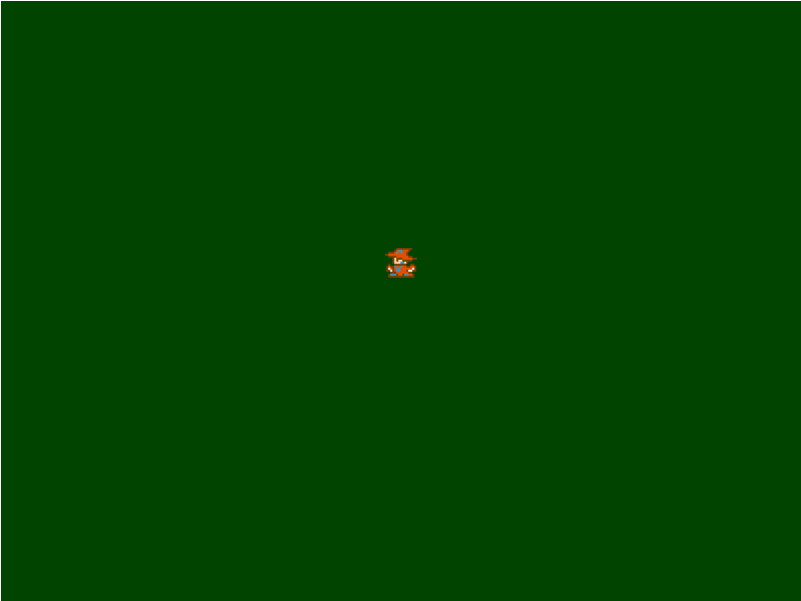# JavaScript and HTML5 – Simple Game Creation Tutorial (part 2a)

All of these changes are now incorporated into part 2 of the Simple Game tutorial

A slight errata is being made to the JavaScript code for part 2 of the Simple Game tutorial. I’ve had it pointed out to me by several people that the way the arrow keys interact with the hero character isn’t very intuitive. To remedy that we’re going to adjust the hero update function to take the most recent key that is pressed as the “active direction” for movement. Because of the way we are capturing keydown and keyup there are no required changes to that section of the code.

What we’ll do is change it from a series of if / else if conditional checks to a simple switch statement using the last key that was pushed into the array. Since we use push and splice to work with the array and we are always checking to see if a key is already in the array or not, we can be sure that the key code in the last index of the array will be the key that was most recently pressed down. Also, I’m going to take this opportunity to adjust the logic that updates the sprite. Previously, we were just doing a check of [cciel_javascript]if (delta > this.animSpeed)[/cciel_javascript] to determine if we should update the sprite that we are displaying or not. This had the unfortunate side effect of not doing an immediate transition on the sprite when the hero changed directions. The fix for that is fairly straight forward and only requires that we check if the animation timer has elapsed or if the current sprite being shown isn’t one of the two valid sprites for the direction we are moving.

The new code that replaces the part 2 update function is:

[ccel_javascript]
this.update = function(elapsed)
{
var now = Date.now();
// How long has it been since we last updated the sprite
var delta = now – this.lastRender;

// perform a switch statement on the last key pushed into the array
// this allows us to always move the direction of the most recently pressed
// key
switch (this.keys[this.keys.length – 1])
{
case 37:
// move the hero left on the screen
this.x -= this.moveSpeed * elapsed;
// Check if the animation timer has elapsed or if we aren’t using one of the
// two valid sprites for this direction
if (delta > this.animSpeed
|| (this.whichSprite != this.width * 2 && this.whichSprite != this.width * 3))
{
// The sprites for moving left are the 3rd and 4th sprites in the image (0 based index)
this.whichSprite = this.whichSprite == this.width * 2 ? this.width * 3 : this.width * 2;
this.lastRender = now;
}
break;
case 38:
// move the hero up on the screen
this.y -= this.moveSpeed * elapsed;
// Check if the animation timer has elapsed or if we aren’t using one of the
// two valid sprites for this direction
if (delta > this.animSpeed
|| (this.whichSprite != this.width * 6 && this.whichSprite != this.width * 7))
{
// The sprites for moving up are the 7th and 8th sprites in the image (0 based index)
this.whichSprite = this.whichSprite == this.width * 6 ? this.width * 7 : this.width * 6;
this.lastRender = now;
}
break;
case 39:
// move the hero right on the screen
this.x += this.moveSpeed * elapsed;
// Check if the animation timer has elapsed or if we aren’t using one of the
// two valid sprites for this direction
if (delta > this.animSpeed
|| (this.whichSprite != this.width * 4 && this.whichSprite != this.width * 5))
{
// The sprites for moving right are the 5th and 6th sprites in the image (0 based index)
this.whichSprite = this.whichSprite == this.width * 4 ? this.width * 5 : this.width * 4;
this.lastRender = now;
}
break;
case 40:
// move the hero down on the screen
this.y += this.moveSpeed * elapsed;
// Check if the animation timer has elapsed or if we aren’t using one of the
// two valid sprites for this direction
if (delta > this.animSpeed
|| (this.whichSprite != 0 && this.whichSprite != this.width))
{
// The sprites for moving down are the 1st and 2nd sprites in the image (0 based index)
this.whichSprite = this.whichSprite == 0 ? this.width : 0;
this.lastRender = now;
}
break;
}

// This code handles wrapping the hero from one edge of the canvas to the other
if (this.x < -this.width) { this.x = gameW - this.width; } if (this.x >= gameW)
{
this.x = 0;
}
if (this.y < -this.height) { this.y = gameH - this.height; } if (this.y >= gameH)
{
this.y = 0;
}

// This code would cause the edges of the canvas to be a barrier
// if (this.x < 0) // { // this.x += this.moveSpeed * elapsed; // } // if (this.x + this.width >= gameW)
// {
// this.x -= this.moveSpeed * elapsed;
// }
// if (this.y < 0) // { // this.y += this.moveSpeed * elapsed; // } // if (this.y + this.height >= gameH)
// {
// this.y -= this.moveSpeed * elapsed;
// }
};
[/ccel_javascript]The code is update on the demo site in the SimpleGame_2.js file.